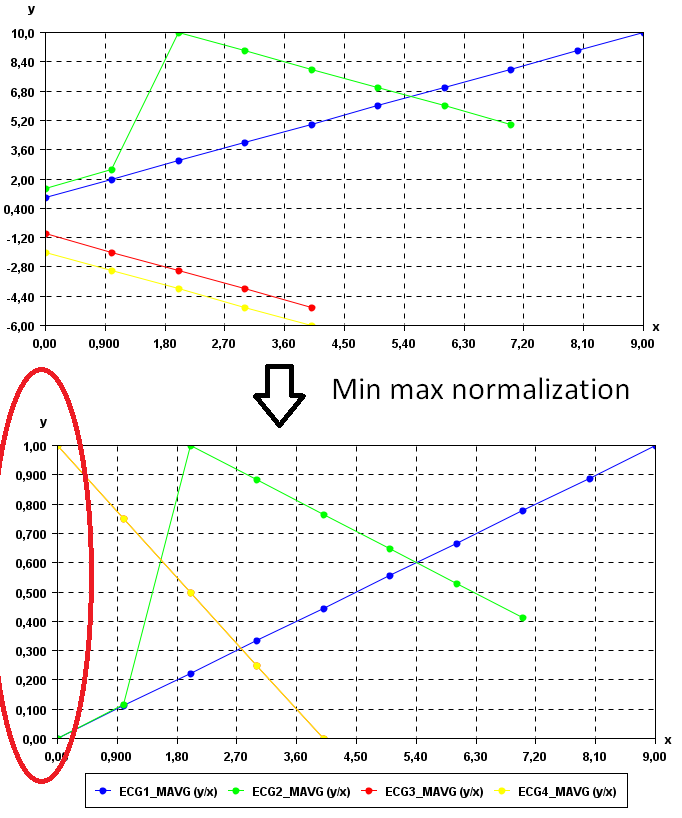# Calculate the min max normalization of time series (SPMF documentation)

This example explains how to calculate the min max normalization of time series using the SPMF open-source data mining library.

## How to run this example?

• If you are using the graphical interface, (1) choose the "Calculate_min_max_normalization_of_time_series" algorithm, (2) select the input file "contextSAX.txt", (3) set the separator to the comma ',' , and then (4) click "Run algorithm".
• If you want to execute this example from the command line, then execute this command:
java -jar spmf.jar run Calculate_min_max_normalization_of_time_series contextSAX.txt output.txt ,
in a folder containing spmf.jar and the example input file contextSAX.txt.
• If you are using the source code version of SPMF, to run respectively this example, launch the file "MainTestMainTestMinMaxNormalizationFileToFile.java"in the package ca.pfv.SPMF.tests.

## What is the calculation of the min max normalization of a time series?

Let there be a time series (a list of data points). Let X and Y be the minimum and maximum values in the time series, respectively. The min max normalization of a time series is obtained by replacing each data point Z by (Z-X)/ (Y-X). That is the min max normalization transform a time series to that all data points appear in the [0,1] interval. This transformation is useful for some data analytic task where it is required that data appears in the [0,1] interval, for example. The output is a time series.

## What is the input of this algorithm?

The input is one or more time series. A time series is a sequence of floating-point decimal numbers (double values). A time-series can also have a name (a string).

Time series are used in many applications. An example of time series is the price of a stock on the stock market over time. Another example is a sequence of temperature readings collected using sensors.

For this example, consider the four following time series:

 Name Data points ECG1 1,2,3,4,5,6,7,8,9,10 ECG2 1.5,2.5,10,9,8,7,6,5 ECG3 -1,-2,-3,-4,-5 ECG4 -2.0,-3.0,-4.0,-5.0,-6.0

This example time series database is provided in the file contextSAX.txt of the SPMF distribution.

In SPMF, to read a time-series file, it is necessary to indicate the "separator", which is the character used to separate data points in the input file. In this example, the "separator" is the comma ',' symbol.

## What is the output?

The output is the min max normalization of the time series received as input. Each original time series is replaced by its min max normalization, calculated as follows.Let there be a time series (a list of data points). Let X and Y be the minimum and maximum values in the time series, respectively. The min max normalization of a time series is obtained by replacing each data point Z by (Z-X)/ (Y-X). That is the min max normalization transform a time series to that all data points appear in the [0,1] interval.

For example, in the above example, the min max normalization of the above four time series is:

 Name Data points ECG1_MMAX 0.0,0.1111111111111111,0.2222222222222222,0.3333333333333333,0.4444444444444444,0.5555555555555556,0.6666666666666666,0.7777777777777778,0.8888888888888888,1.0 ECG2_MMAX 0.0,0.11764705882352941,1.0,0.8823529411764706,0.7647058823529411,0.6470588235294118,0.5294117647058824,0.4117647058823529 ECG3_MMAX 1.0,0.75,0.5,0.25,0.0 ECG4_MMAX 1.0,0.75,0.5,0.25,0.0

To see the result visually, it is possible to use the SPMF time series viewer, described in another example of this documentation. Here is a figure showing the oriignal time series (top) and the resulting min max normalized time series corresponding to these time series.As it can be observed all time series are now in the [0,1] interval.

## Input file format

The input file format is defined as follows. It is a text file. The text file contains one or more time series. Each time series is represented by two lines in the input file. The first line contains the string "@NAME=" followed by the name of the time series. The second line is a list of data points, where data points are floating-point decimal numbers separated by a separator character (here the ',' symbol).

For example, for the previous example, the input file is defined as follows:

@NAME=ECG1
1,2,3,4,5,6,7,8,9,10
@NAME=ECG2
1.5,2.5,10,9,8,7,6,5
@NAME=ECG3
-1,-2,-3,-4,-5
@NAME=ECG4
-2.0,-3.0,-4.0,-5.0,-6.0

Consider the first two lines. It indicates that the first time series name is "ECG1" and that it consits of the data points: 1, 2, 3, 4, 5, 6, 7, 8, 9, and 10. Then, three other time series are provided in the same file, which follows the same format.

## Output file format

The output file format is the same as the input format. For example, there is the result of this example:

@NAME=ECG1_MMAX
0.0,0.1111111111111111,0.2222222222222222,0.3333333333333333,0.4444444444444444,0.5555555555555556,0.6666666666666666,0.7777777777777778,0.8888888888888888,1.0
@NAME=ECG2_MMAX
0.0,0.11764705882352941,1.0,0.8823529411764706,0.7647058823529411,0.6470588235294118,0.5294117647058824,0.4117647058823529
@NAME=ECG3_MMAX
1.0,0.75,0.5,0.25,0.0
@NAME=ECG4_MMAX
1.0,0.75,0.5,0.25,0.0